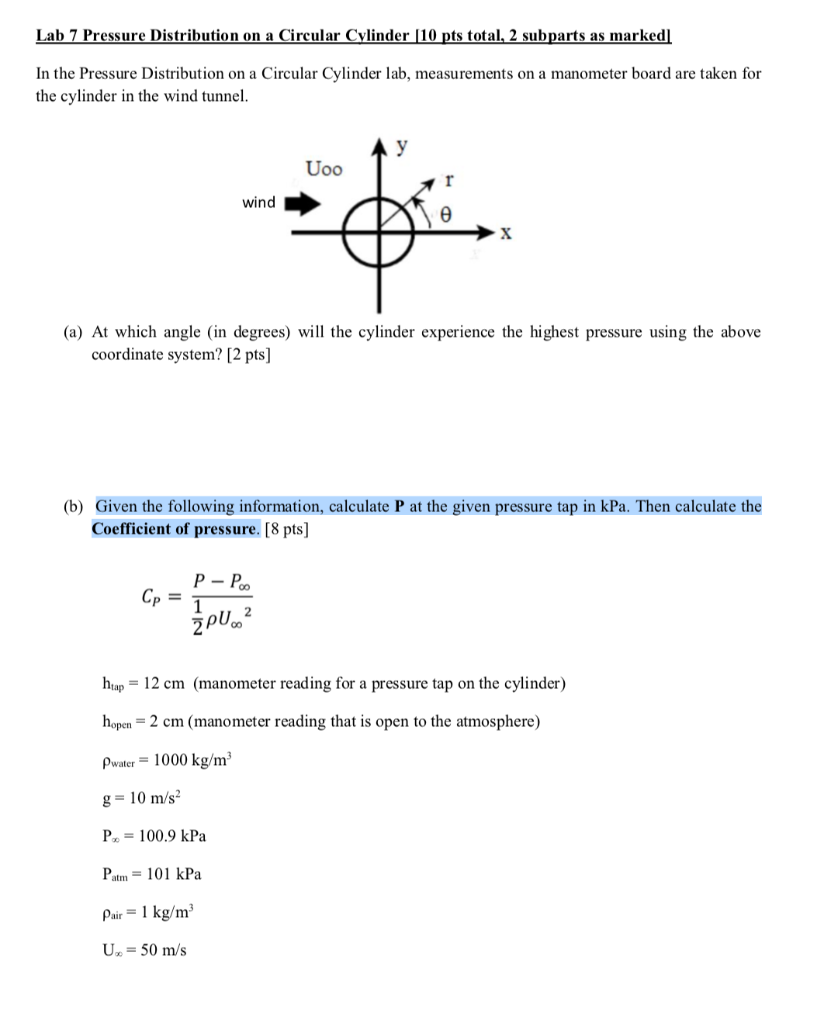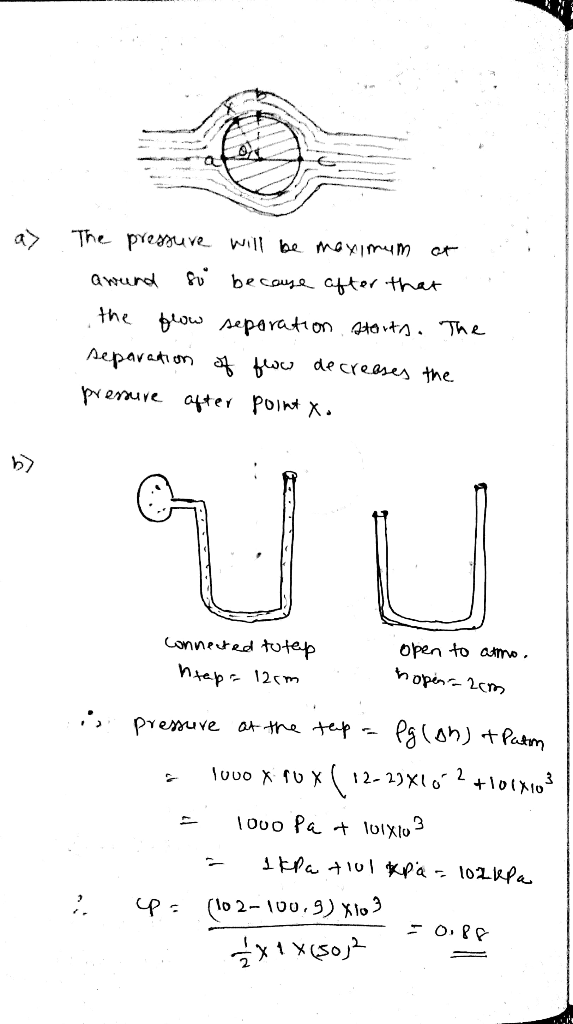Homework Help Question & Answers

Lab 7 Pressure Distribution on a Circular Cvlinder [10 pts total, 2 subparts as marked In the Pre...Lab 7 Pressure Distribution on a Circular Cvlinder [10 pts total, 2 subparts as marked In the Pressure Distribution on a Circular Cylinder lab, measurements on a manometer board are taken for the cylinder in the wind tunnel wind (a) At which angle (in degrees) will the cylinder experience the highest pressure using the above coordinate system? [2 pts] (b) Given the following information, calculate P at the given pressure tap in kPa. Then calculate the Coefficient of pressure. [8 pts] 2 hap-12 cm (manometer reading for a pressure tap on the cylinder) hopen- 2 cm (manometer reading that is open to the atmosphere) water 1000 kg/m g=10m/s, 100.9 kPa Patm- 101 kPa Pair 1 kg/m3 U 50 m/sAdd Answer of: Lab 7 Pressure Distribution on a Circular Cvlinder [10 pts total, 2 subparts as marked In the Pre...
More Homework Help Questions Additional questions in this topic.

• [2 pts] in decimal, what does b-? a. short a --1 ushort *b &a b-? [2 pts] What conditions are required for a race condition? b. [2 pts] The purpose of software security is? c. [2 pts] in...

Need Online Homework Help?

Get FREE EXPERT Answers
WITHIN MINUTES
Related Questions